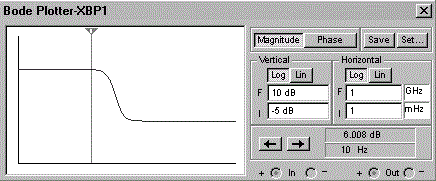# Electronics - Capacitors

### Exercise :: Capacitors - General Questions

26.

In a capacitive circuit, current flow is limited to

 A. charging periods B. discharging periods C. neither charging periods nor discharging periods D. both charging periods and discharging periods

Explanation:

No answer description available for this question. Let us discuss.

27.

What circuit has the following frequency response?A. bandpass filter B. high-pass filter C. low-pass filter D. integrator

Explanation:

No answer description available for this question. Let us discuss.

28.

A capacitor is constructed of two parallel:

 A. conductors B. semiconductors C. inductors D. dielectrics

Explanation:

No answer description available for this question. Let us discuss.

29.

Which of the following determines the capacitance of a capacitor?

 A. Plate area, dielectric strength, and plate separation B. Voltage rating, dielectric constant, and temperature coefficient C. Temperature coefficient, plate area, and plate separation D. Plate area, dielectric constant, and plate separation

Explanation:

No answer description available for this question. Let us discuss.

30.

Most older types of paper capacitors have been replaced by which type of construction?

 A. electrolytic B. plastic film C. oxide casing D. waxed paper